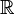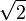# Real number facts for kids

Kids Encyclopedia Facts

A real number is a rational or irrational number, and is a number which can be expressed using decimal expansion. Usually when people say "number", they usually mean "real number". The official symbol for real numbers is a bold R, or a blackboard bold$\mathbb{R}$.

Some real numbers are called positive. A positive number is "bigger than zero". Real numbers can be thought of as an infinitely long ruler. There is a mark for zero and every other number, in order of size. Unlike a physical ruler, there are numbers below zero. These are called negative real numbers. Negative numbers are "smaller than zero". They are like a mirror image of the positive numbers, except that they are given minus signs (–) so that they are labeled differently from the positive numbers.

There are infinitely many real numbers, because there is no smallest or biggest real number. No matter how many real numbers are counted, there are always more which need to be counted. There are no empty spaces between real numbers, which means that if two different real numbers are taken, there will always be a third real number between them. This is always true no matter how close together the first two numbers are.

If a positive number is added to another positive number, then that number gets bigger. If zero, which is also a real number, is added to a number, then that number does not change. If a negative number is added to another number, that number gets smaller.

The real numbers are uncountable, which means that there is no way to put all the real numbers into a sequence. Any sequence of real numbers will miss out a real number, even if the sequence is infinite. This makes the real numbers special, because even though there are infinitely many real numbers and infinitely many integers, there are "more" real numbers than integers. This is often expressed by saying that the integers are countable and that the real numbers are uncountable.

Some simpler number systems are inside the real numbers. For example, the rational numbers and integers are all in the real numbers. There are also more complicated number systems than the real numbers, such as the complex numbers. Every real number is a complex number, but not every complex number is a real number. Similarly, 3/7 is a rational number but not an integer.

## Different types of real numbers

There are different types of real numbers. Sometimes all the real numbers are not talked about at once. Sometimes only special, smaller sets of them are talked about. These sets have special names. They are:

• Natural numbers: These are real numbers that have no decimal and are bigger than zero.
• Whole numbers: These are positive real numbers that have no decimals, and also zero. Natural numbers are also whole numbers.
• Integers: These are real numbers that have no decimals. These include both positive and negative numbers. Whole numbers are also integers.
• Rational numbers: These are real numbers that can be written as fractions of integers. Integers are also rational numbers.
• Transcendental numbers cannot be obtained by solving an equation with integer components.
• Irrational numbers: These are real numbers that can not be written as a fraction of integers. Transcendental numbers are also irrational.

The number 0 (zero) is special. Sometimes it is taken as part of the subset to be considered, and at other times it is not. It is the Identity element for addition, which means that adding zero does not change the original number. For multiplication, the identity element is 1.

One real number that is not rational is$\sqrt{2}$. This number is irrational. If a square is drawn with sides that are one unit long, then the length of the line between its opposite corners will be$\sqrt{2}$.

## Related pagesIn Spanish: Número real para niños